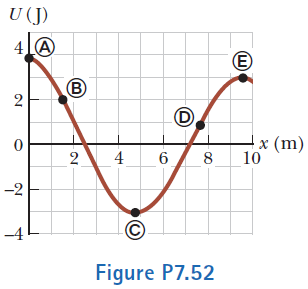# Problem: For the potential energy curve shown in Figure P7.52, (a) determine whether the force Fx is positive, negative, or zero at the five points indicated. (b) Indicate points of stable, unstable, and neutral equilibrium. (c) Sketch the curve for Fx versus x from x = 0 to x = 9.5 m.

⚠️Our tutors found the solution shown to be helpful for the problem you're searching for. We don't have the exact solution yet.

###### Problem Details

For the potential energy curve shown in Figure P7.52, (a) determine whether the force Fx is positive, negative, or zero at the five points indicated. (b) Indicate points of stable, unstable, and neutral equilibrium. (c) Sketch the curve for Fx versus x from x = 0 to x = 9.5 m.